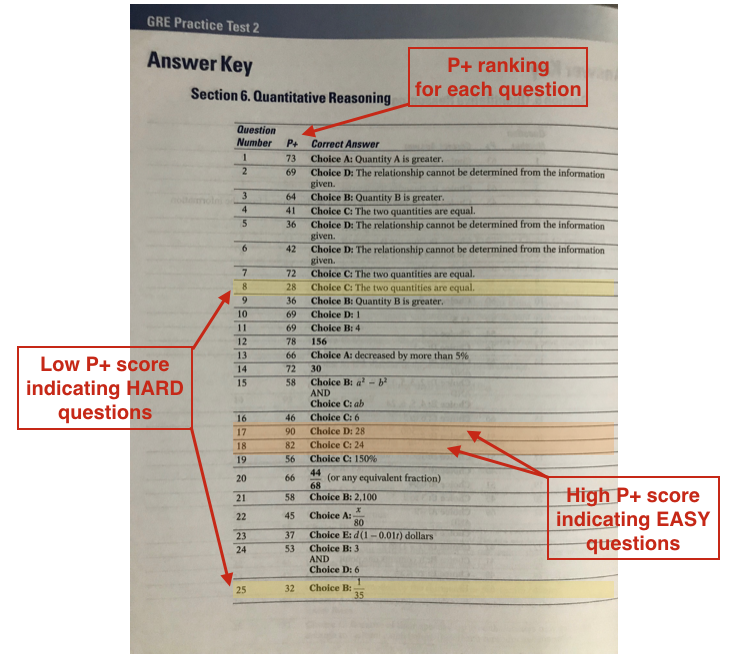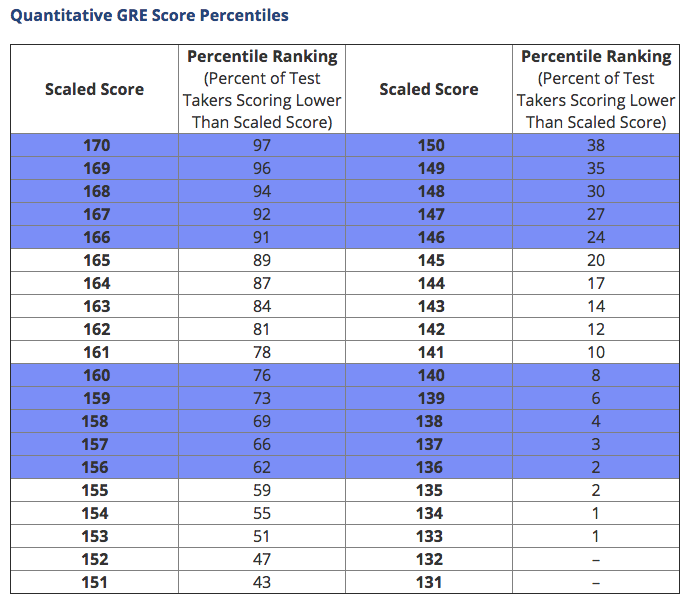Over Age 30 and Taking the GRE? Learn These 3 Key Things!   Get the FREE Video!A question I occasionally get from my students goes something like this:

How many questions do I need to get right on the quant/verbal section to get a score of __________?

If only it were that simple.

Remember that the GRE is a computer-adaptive test. More specifically, it’s section-adaptive meaning that it adapts to your abilities and gives you subsequent sections that contain either harder or easier questions depending on how you’re doing. As such, each test taker’s score path is unique and the number of correct answers doesn’t perfectly correlate to a specific score. Consider the following two hypothetical GRE candidates.

Person A: Got 16 out of 20 questions correct on the first quantitative section. Was given a significantly harder second quantitative section. Got 11 out of 20 questions correct on that second quantitative section.

Person B: Got 12 out of 20 question correct on the first quantitative section. Was given a moderately easier second quantitative section. Got 15 out of 20 questions correct on that second quantitative section.

Both test takers got 27 correct answers out of 40 total questions. Yet, their respective Quant scores on the 130-170-point scale would not be the same because they didn’t see questions of the same difficulty level throughout. While the exact scoring algorithm used by ETS to calculate scaled scores is not made public, anecdotal evidence suggests that Person A would likely get a higher score than Person B for having “elevated” to a harder second section.

The point is that it’s not as easy as simply looking at the number of correct answers as a predictor of your score. So what should you do if you’re trying to work toward a target GRE score? Is there anything you can look at during your practice sessions to gauge your progress?

I propose using the P+ rating in the Official Guide to the GRE publications as a useful metric.

## Understanding P+

The Official Guide to the GRE is an indispensable resource when preparing for the GRE because it’s the only book with sample questions straight from the makers of the GRE. That’s why I assign practice problems from it in my online GRE prep courses.

What’s particularly helpful is a little-known and even less-understood scoring metric called “P+” that appears in the answer key for each of the two practice tests at the back of the book. The P+ is the percent of a group of actual GRE takers who were administered that same question at a previous test administration and who answered it correctly. P+ is used to gauge the relative difficulty of a test question.

Here’s what that means. Take a look at this answer key for Section 6 of GRE Practice Test 2 from the 3rd edition of The Official Guide to the GRE:First, notice the column between the question numbers and correct answer explanations. That’s the P+. There’s one for each question.

In terms of interpreting P+, look at question 17. It has a P+ of 90. That means that 90% of GRE test takers who previously answered this question got it right. That’s almost everyone. In other words, it’s an easy question.

By contrast, look at question 8. It has a P+ of 28, meaning that only 28% of respondents got that question correct. That’s not very many. Question 8 is therefore a hard question.

See how that works? And do you see how helpful that can be in evaluating your level of preparedness for the real GRE? Let’s take it a step further and look at how you can use P+ to make sure you’re on track for your target GRE score.

## Reconciling P+ with GRE Percentile Rankings

Now that you understand P+, let’s revisit the concept of percentile rankings. If you haven’t done so yet, read my article on GRE percentile rankings before going any further. For convenience, here’s the percentile ranking table for GRE quant (check out my article if you want the percentile ranking charts for verbal and AWA).As a quick refresher, when you receive your official GRE score you will see both your scaled score (on a scale from 130 – 170) and its percentile ranking. You can think of a percentile ranking as the percent of other test takers worldwide who scored worse than that given score. For example, a GRE Quantitative score of 159 has a percentile ranking of 73. That means that with a score of 159 on quant you will have scored higher than 73% of other test takers worldwide. Not bad!

So how is this relevant to P+ in terms of predicting your real GRE score?

It’s pretty simple. P+ and percentile rankings are essentially inverses of each other. Let’s assume your target GRE quant score is in fact 159. You now know that means you’ll have to score higher than 73% of all other test takers. Now here’s the fun part. Another way to think about that is, you need to be able to answer questions than only 27% of test takers are able to get right. That’s the P+. Pretty cool, huh?

Let’s look at another example. What if your target quant score is only 150? That score will place you in the 38th percentile, meaning you need to score higher than 38% of all other test takers. Put another way, you need to be able to get right answers on questions that 62% (or more) of test takers are able to get right. So now look back at the answer key from Section 6 of GRE Practice Test 2 (above). The following questions have a P+ of 62 or higher:

• Question 1 (P+ 73)
• Question 2 (P+ 69)
• Question 3 (P+ 64)
• Question 7 (P+ 72)
• Question 10 (P+ 69)
• Question 11 (P+ 69)
• Question 12 (P+ 78)
• Question 13 (P+ 66)
• Question 14 (P+ 72)
• Question 17 (P+ 90)
• Question 18 (P+ 82)
• Question 20 (P+ 66)

So if that’s you and you want to make sure you’re on track to score a 150 on GRE Quant, simply go to the practice tests at the back of the Official Guide and make sure you can answer all of the questions with a P+ of 62 or higher!

You can obviously use this strategy for whatever your target GRE score is.

Oh, and incidentally, notice that you would have gotten 12 out of 20 questions right on Section 6 assuming you got every question with a P+ of 62 or higher correct. Theoretically, then, 12/20 right answers would correspond to a GRE Quant score of 150 based on the level of difficulty of questions on this particular section. But as we noted earlier, some sections may have more or fewer questions with a P+ greater than 62, so you may need to get more or fewer than 12 right answers per section to lock in your 150.

In any event, predicting your GRE score isn’t an exact science, but hopefully you now see the value of using P+ to make sure you’re on track for geting your target GRE score on test day.